# Basic Geometry : How to find a ray

## Example Questions

← Previous 1

### Example Question #1 : Lines

Which of the following are the sides of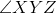?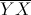and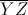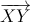and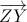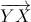and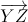and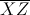andandExplanation:

The sides of an angle are two rays, both of which begin at a common vertex. Since the middle letter of the name of this angle is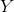is the common endpoint; therefore, the name of each ray starts with. This makes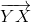and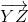the correct choice.

Note that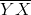and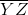cannot be correct: these are line segments.

### Example Question #1 : How To Find A Ray

A ray is different from a line in that

A line can be curved but a ray is always straight

A line has a beginning and a ray does not

A ray can cross itself

One side of the ray goes on forever while the other stops

One side of the ray goes on forever while the other stops

Explanation:

A ray is defined by two points, one that defines the beginning, and the other that defines the direction - this ray goes on forever in whatever direction is defined by that second point

### Example Question #1 : How To Find A Ray

Which of the following is not a side of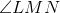?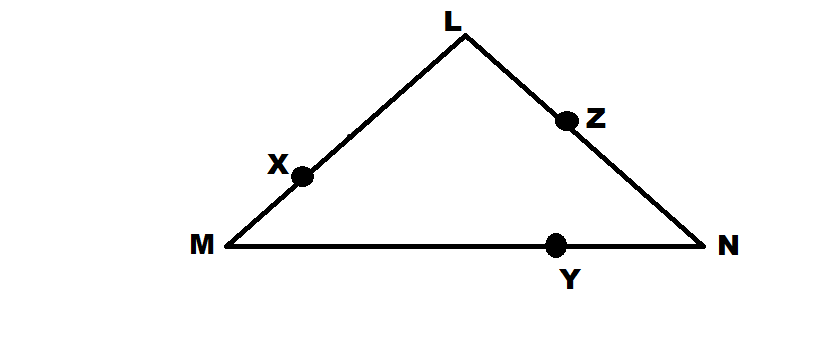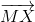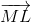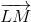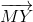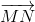Explanation:

The two sides of an angle are the two rays that compose it. Each of these rays begins at the vertex and proceeds out from there. In naming a ray, we always begin with the letter of the endpoint (where the ray starts) followed by another point on the ray in the direction it travels. Since the vertex of the angle is the endpoint of each ray and our vertex is, each of our rays must begin with. Onlyfails to do so.

### Example Question #4 : Lines

How does a ray differ from a line segment?

A line segment continues in both directions, a ray only continues in one direction.

A line segment has two end points, a ray only has one.

Ray has two end points.

A ray has two end points whereas a line segment only has one.

A line segments connects to itself forming a shape, a ray does not.

A line segment has two end points, a ray only has one.

Explanation:

Line segment: part of a line that has two distinct end points.

Ray: portion of a line with one end point, where the other end of the line continues to infinity.

### Example Question #1 : How To Find A Ray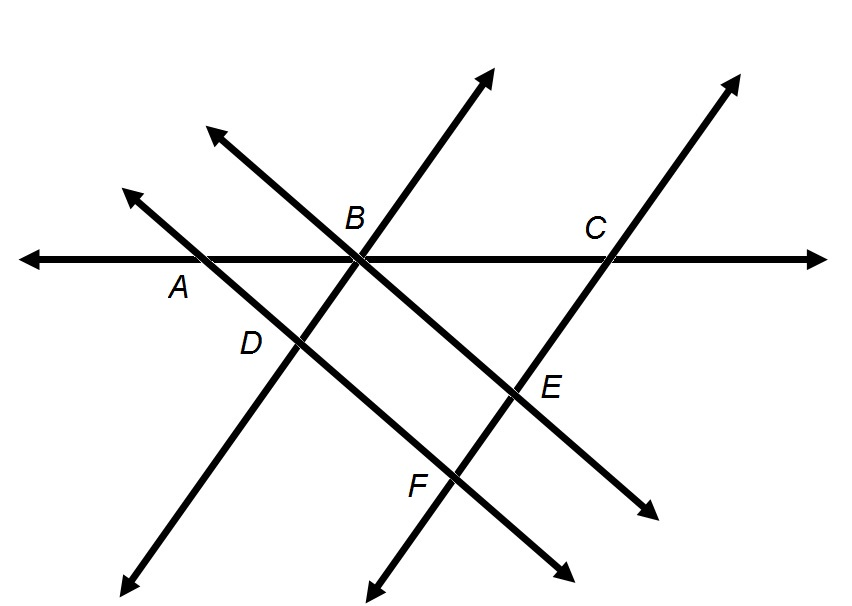Refer to the above diagram. The plane containing the above figure can be called Plane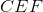.

True

False

False

Explanation:

A plane can be named after any three points on the plane that are not on the same line. As seen below, points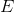,  andare on the same line.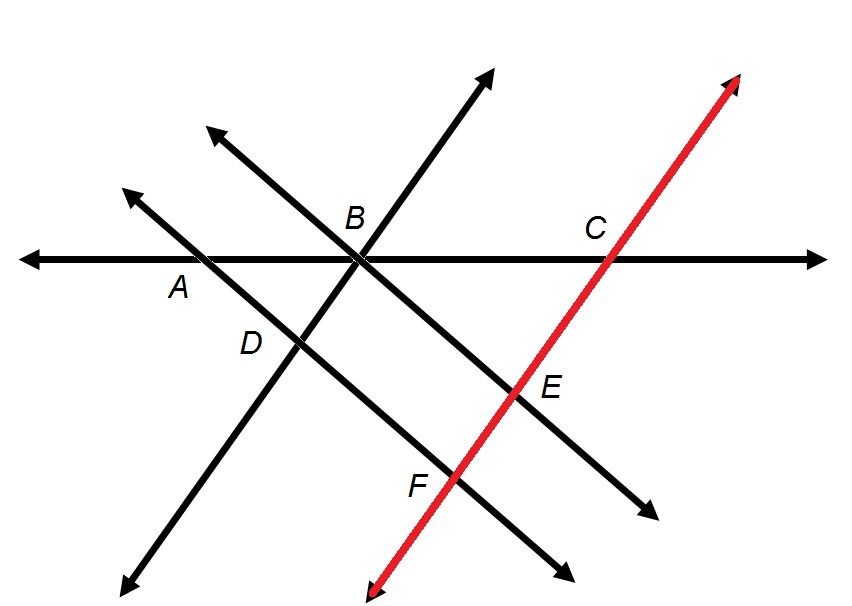Therefore, Planeis not a valid name for the plane.

### Example Question #3 : How To Find A RayRefer to the above diagram.

True or false: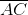can also be called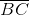.

True

False

False

Explanation:

A line segment has two letters in its name, each of which is an endpoint.is therefore the segment marked in red below.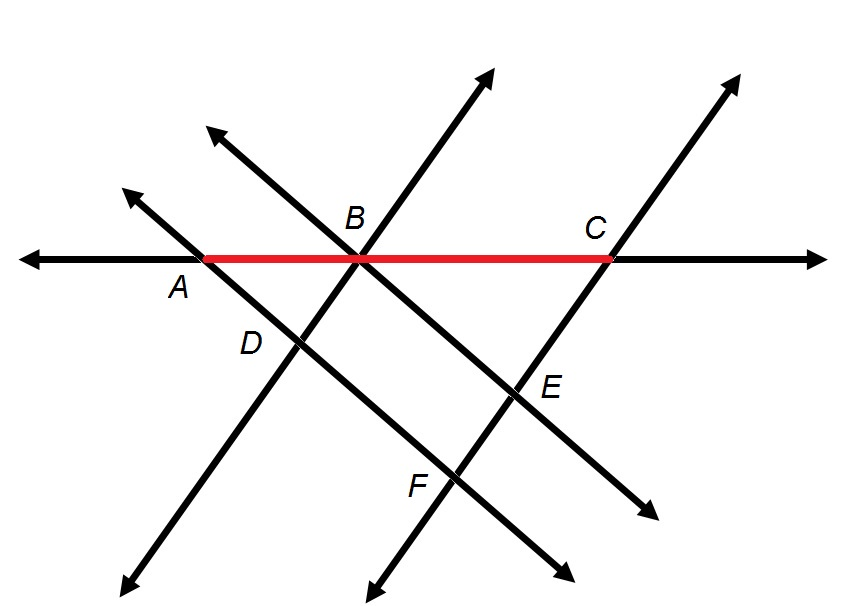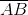is not a valid name, sinceis not an endpoint of the segment.

### Example Question #7 : LinesRefer to the above diagram.

True or false:can also be called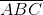.

False

True

False

Explanation:

A segment is named with only two letters, each of which is an endpoint.is not a valid name.

### Example Question #4 : How To Find A RayRefer to the above diagram.

True or false:and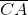refer to the same line segment.

False

True

True

Explanation:

The two letters in the name of a line segment are its endpoints in either order. Therefore,is the segment with endpointsand; consequently, it can also be called.

### Example Question #9 : LinesRefer to the above diagram.

True or false: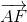and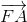refer to the same ray.

True

False

False

Explanation:

A ray is named with two letters, the first of which is an endpoint and the second of which is any other point the ray passes through.is a ray with endpoint, andis a ray with endpointandare therefore not the same ray.

### Example Question #2 : How To Find A RayRefer to the above diagram.

True or false: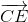and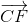refer to the same ray.

False

True

True

Explanation:

A ray is named with two letters, the first of which is an endpoint and the second of which is any other point the ray passes through.has its endpoint atand passes through; it is the ray marked in red in the figure below: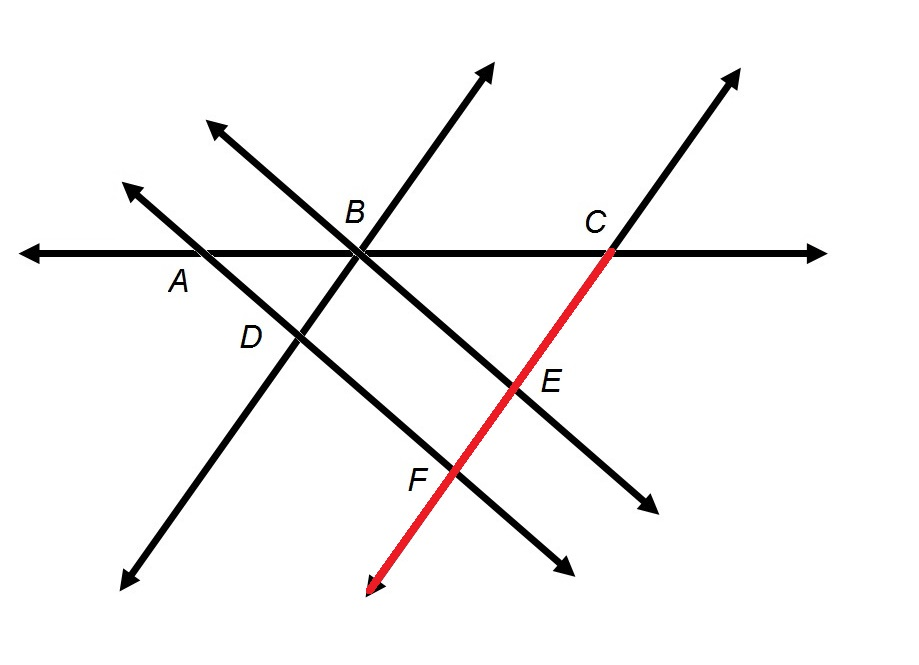The ray also passes through Point, so the ray can also be called.

← Previous 1

### All Basic Geometry Resources